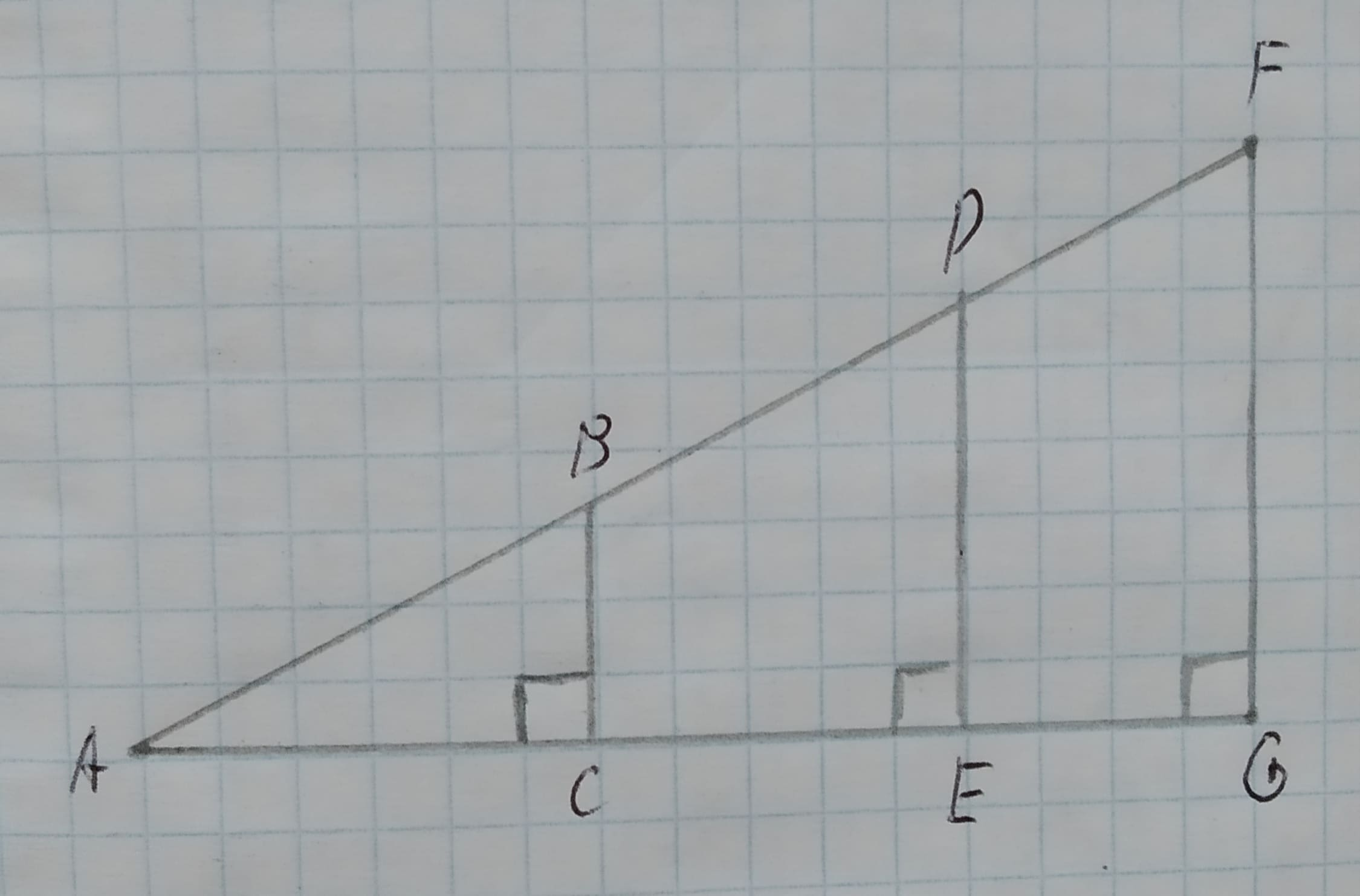# Similar Triangles and Triginimetric Functions Use the figure below. What does similarity imply about the ratios (BC)/(AB), (DE)/(AD), and (FG)/(AF)?avissidep 2021-03-09 Answered
Similar Triangles and Triginimetric Functions Use the figure below.
What does similarity imply about the ratios $\frac{BC}{AB},\frac{DE}{AD},\phantom{\rule{1em}{0ex}}\text{and}\phantom{\rule{1em}{0ex}}\frac{FG}{AF}$?
You can still ask an expert for help

• Questions are typically answered in as fast as 30 minutes

Solve your problem for the price of one coffee

• Math expert for every subject
• Pay only if we can solve itmhalmantus

Given:
Consider the given triangle,Calculation:
From part (a), the given triangles are similar.
In similar triangles, the ratio of the corresponding sides is equal.
$\frac{BC}{AB}=\frac{DE}{AD}=\frac{FG}{AF}$
Therefore, from the given triangles,
Hence, the given ratios are equal.